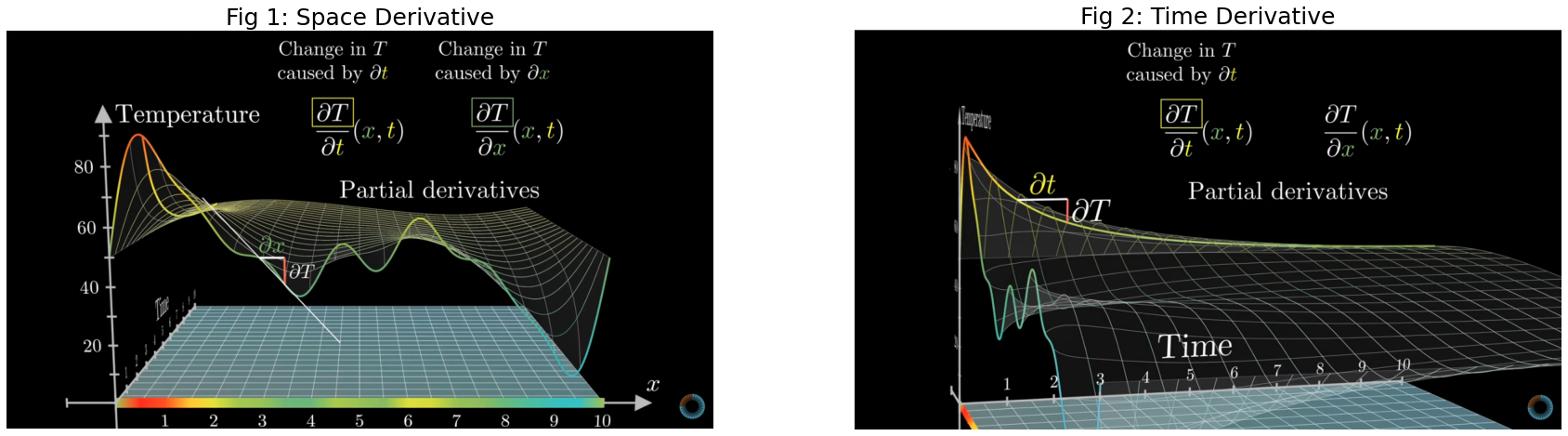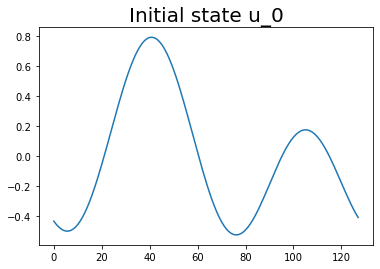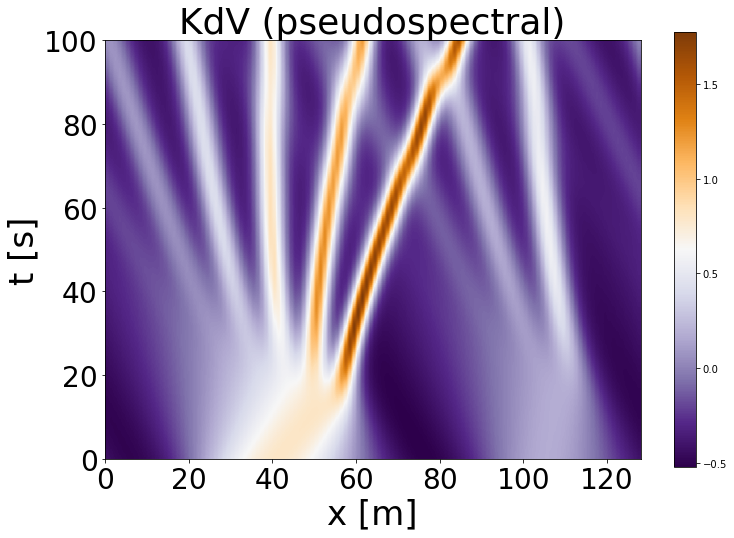# DNN - Tutorial 2 Part II: Physics inspired Machine Learning¶

Partial Differential Equation (PDE) Solvers

Before we start one practical thing:

Later in this tutorial we will use datasets to train our own Neural PDE Solver. It takes a little bit of time for the datasets to be uploaded, so let’s do that first! (So by the time you get to the coding part the data is already in your environment)

• Go to: drive

• Upload them here: click on ‘Files’ –> right click ‘Upload’ (click ‘ok’ on the prompt)

## PDE vs ODE: What are PDEs?¶

A partial derivative of a function (with several variables) is a derivative with respect to one of its variables while the other variables are held constant.

For example take the Heat equation (1 dimension):

$\frac{\delta T}{\delta t}(x,t)=\frac{\delta^{2}T}{\delta x^{2}}(x,t)$

Here we have one partial derivative w.r.t time (left hand side, LHS), and another partial derivative w.r.t to space (right hand side, RHS), as our function T(x,t) has 2 variables. On the LHS variable $$x$$ is held constant, while on the RHS variable $$t$$ is held constant.

In particular, the above PDE describes how the temperature T(x,t) changes in time for a 1 dimensional object, such as a metal rod. If we look at the PDE equation above it says that the rate of change of temperature in time is the same as the the second order derivate of the spatial domain.

This makes sense as the second order derivate gives you a measure of how a value compares to the average of its neighbours. For example, if the neighbors of a given point $$x_{i}$$ are very cold compared to the point itself then the rate of change in temperature in time for this point will be faster.

In the image below we have visualized the partial derivatives of the 1d Heat equation. We can investigate the rate of change of the temperature in two domains: spatial (see Fig 1) and time (see Fig 2). (credits: 3Blue1Brwon series source)

:

import matplotlib.pyplot as plt
import matplotlib.image as mpimg

f, axarr = plt.subplots(1,2, figsize=(30,30))
axarr.set_axis_off()
axarr.set_title('Fig 1: Space Derivative', fontsize=25)
axarr.set_axis_off()
axarr.set_title('Fig 2: Time Derivative', fontsize=25)
axarr.imshow(img_space)
axarr.imshow(img_time);As you can see above each of the derivatives tells only a part of the story on how the function changes, therefore we call them partial derivates. In principle, PDEs could be seen as a system of ordinary differential equations.

In dynamical systems compared to traditional ML we are most interested in how our function T(x,t) changes over time. The above 1D Heat equation can be also extended to more spatial directions by extending the right hand side:

$\frac{\delta T}{\delta t}(x,y,z,t) = \alpha * (\frac{\delta^{2}T}{\delta x^{2}}(x,y,z, t) + \frac{\delta^{2}T}{\delta y^{2}}(x,y,z, t) + \frac{\delta^{2}T}{\delta z^{2}}(x,y,z, t))$

Why do we care about PDEs?

Thay allow us to model (predict) real life systems that influence our life: - economics: Black-Scholes equation that governs price evolution - climate: Wheather prediction, think about how your google weather app knows what’s the weather going to be like tomorrow - in general, the entire physical world around us

To conclude:

PDEs tells us how a function with several variables changes over time. Most of the physical world is governed by PDEs.

## How to solve PDEs?¶

Let’s now shift our focus on a specfic PDE, for example, the Korteweg-de Vries (KdV) equation.

The KdV euqation (1985) is a model of shallow water waves:In the above x again represents a spatial location, U(x) is the value of x given a function U, and we can see the evolution of the system in time.

The PDE equation governing the above simulation is:

$\frac{\delta U}{\delta t}(x,t) = - U(x,t) * \frac{\delta U}{\delta x}(x,t) - \frac{\delta^{3}U}{\delta x^{3}}(x,t)$

Similar to the heat equation in the introduction our function U has two dimentions, x and t, where x is the spatial domain and t is the time domain. The $$\frac{\delta^{3}U}{\delta x^{3}}(x,t)$$ is a dispersive term and $$U(x,t) * \frac{\delta U}{\delta x}(x,t)$$ is a non-linear convection term.

When we are taking about solving a PDE what we want to do is to find a function U(x,t) that would: - satisfy the above given PDE - meet the boundary conditions - meet the initial conditions

These additional constraints, boundary and inital conditions, restrict the function U(x,t) that is valid for the given PDE equation

Boundary Conditions

The boundary conditions express the behaviour of a function on the boundary (border) of its area of definitions. For example, you constrain that at both ends of the spatial domain $$x_{0}$$ and $$x_{L}$$ (where L is the end of your spatial domain) the function remains constant for all time:

$\frac{\delta U}{\delta x}(x_{0},t)=\frac{\delta U}{\delta x}(x_{L},t)=0$
$\forall t$

Initial Conditions

An initial condition is similar to boundary conditions, but now in the time direction. For example, now you constrain what value your function U(x,t) must return at timepoint t=0. For the KdV simulation above the initial condition was:

$$U(x,0) = cos(\pi x)$$ $$\forall x$$

Solving PDE

Similary to ODEs the traditional way of solving a given PDE equation is via numerical solvers.

In the below cells we will implement a simple numerical solver in order to solve the above KdV equation

### Numerical Solvers¶

:

#Import relevant packages
%matplotlib inline
import matplotlib
from matplotlib.pyplot import cm
import numpy as np
from typing import Optional
from scipy.integrate import solve_ivp
from scipy.fftpack import diff as psdiff

from matplotlib import animation
import seaborn as sns
from IPython.display import HTML


#### Generate Initial Conditions¶

Initial conditions are sampled from a distribution over truncated Fourier series with random coefficients $$\{A_k, \ell_k, \phi_k\}_k$$ as

$U(x,0) = \sum_{k=1}^N A_k \sin(2 \pi \ell_k x / L + \phi_k) \ .$
:

def generate_params() -> (int, np.ndarray, np.ndarray, np.ndarray):
"""
Returns parameters for initial conditions.
Args:
None
Returns:
int: number of Fourier series terms
np.ndarray: amplitude of different sine waves
np.ndarray: phase shift of different sine waves
np.ndarray: frequency of different sine waves
"""
N = 10 #Number of different waves
lmin, lmax = 1, 3 #sine frequencies for intial conditions
A = (np.random.rand(1, N) - 0.5)
phi = 2.0*np.pi*np.random.rand(1, N)
l = np.random.randint(lmin, lmax, (1, N))
return (N, A, phi, l)

def initial_conditions(x: np.ndarray, L: int, params: Optional[list]=None) -> np.ndarray:
"""
Return initial conditions based on initial parameters.
Args:
x (np.ndarray): input array of spatial grid
L (float): length of the spatial domain
params (Optinal[list]): input parameters for generating initial conditions
Returns:
np.ndarray: initial condition
"""
if params is None:
params = generate_params()
N, A, phi, l = params
u = np.sum(A * np.sin((2 * np.pi * l * x[:, None] / L ) + phi), -1)
return u



#### Solve via Method of Lines (MOL)¶

In MOL all but the temporal dimension are discretized. Having the spatial derivatives numerically implemented results in a set of coupled ODEs for the time domain, which can be solved by using integration schemes of ODE solving.

Concretely, for getting numerical spatial derivatives, we use pseudospectral methods, where the derivatives are computed in the frequency domain by first applying a fast fourier transform (FFT) to the data, then multiplying by the appropriate values and converting back to the spatial domain with the inverse FFT.

Mathematically this works since the Fourier transform of the $$n$$th derivative is given by:

$\widehat{f^{(n)}} (\xi) = \mathcal{F} \bigg( \frac{d^n}{dx^n} f(x) \bigg) = (2\pi i \xi)^n \widehat{f}(\xi) \ ,$

where $$\widehat{f^{(n)}}$$ is the mathematical denotation of fourier transform of a function $$f$$, the transform variable $$\xi$$ represents frequency, $$f(x)$$ is an absolutely continuous differentiable function, and both $$f$$ and its derivative $$f′$$ are integrable (for more information see wikipedia section Functional relations). This method of differentiation in the Fourier space is implemented by the diff function in the module scipy.fftpack. For integration in time we use an implicit Runge-Kutta method of Radau IIA family, order 5.

:

# Spatial Derivatives
def kdv_pseudospectral(t: float, u: np.ndarray, L: float) -> np.ndarray:
"""
Compute spatial derivatives for the KdV equation, using a pseudospectral method, descretization in x.
Args:
t (float): time point
u (np.ndarray): 1D input field
L (float): length of the spatial domain
Returns:
np.ndarray: reconstructed pseudospectral time derivative
"""
# Compute the x derivatives using the pseudo-spectral method.
ux = psdiff(u, order=1, period=L)
uxxx = psdiff(u, order=3, period=L)
# Compute du/dt.
dudt = -u*ux - uxxx
return dudt



#### Solve for KdV trajectory¶

:

# Set the size of the domain, and create the discretized grid.
np.random.seed(1)
L = 128
N = 2**7
x = np.linspace(0, (1-1.0/N)*L, N)

# Set the tolerance of the solver
tol = 1e-6

# Set the initial conditions.
u0 = initial_conditions(x, L)

# Set the time sample grid.
T = 100.
t = np.linspace(0, T, 200)

# Compute the solution using kdv_pseudospectral as spatial solver
sol_ps = solve_ivp(fun=kdv_pseudospectral,
t_span=[t, t[-1]],
y0=u0,
t_eval=t,
args=(L,),
atol=tol,
rtol=tol)


#### Visualize the solved KdV¶

Let’s first plot our initial state:

:

plt.plot(u0)
plt.title('Initial state u_0', fontsize=20)
plt.show();Now let’s plot the entire time evolution, where time is as the y axis, spatial domain as the x axis, and the function value U(x,t) is the colorbar

:

# Let's look at the trajectory obtained by using the pseudospectral spatial solver
if sol_ps.success:
t_ps = sol_ps.y.T[::-1]
plt.figure(figsize=(12,8))
plt.imshow(t_ps, extent=[0,L,0,T], cmap='PuOr_r')
plt.colorbar()
plt.title('KdV (pseudospectral)', fontsize=36)
plt.xlabel('x [m]', fontsize=34)
plt.ylabel('t [s]', fontsize=34)
plt.yticks(fontsize=28)
plt.xticks(fontsize=28)
plt.show()In the figure above we can see the time evolution of our initial state $$U(x,t_{0})$$. As we only have one spatial domain, each $$x$$ point is a coordinate in our spatial domain, for example, the bottom left point would be the value of $$U(x_{0},t_{0})$$. The color indicates the value of our function $$U(.)$$, and the more brown the color the higher the wave. Interpret the stripes you see as waves and as time progresses the waves get sharper.

Feel free to play around with the settings under generate_params() and see how the simulation changes!

Let’s create our own animation!

:

plt.rcParams["animation.html"] = "jshtml"

:

fig, ax = plt.subplots(figsize=(5,5))
t_ps_anim = np.flipud(t_ps)

def animate(t):
plt.cla()
plt.ylim([-0.8, 1.5])
plt.plot(t_ps_anim[t,:])

matplotlib.animation.FuncAnimation(fig, animate, frames=200, interval=20)

: